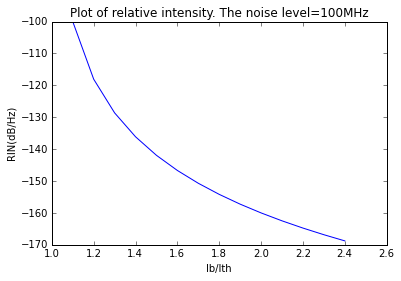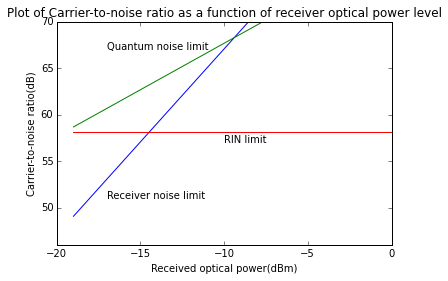Example 9.1, Page Number: 320¶

In :
import math
import matplotlib.pyplot as plt
import numpy as np
import scipy as sp
from scipy import special

%matplotlib inline

#variable decalration
ib_by_ith1=1.5                         #injection current1 > 1.2
ib_by_ith2=1.6                         #injection current2
ib_by_ith3=1.7                         #injection current3
f = 100.0*10**6                          #frequency (hz)

#calculation
RIN1 = ((ib_by_ith1-1)**-3)/f
RIN2 = ((ib_by_ith2-1)**-3)/f
RIN3 = ((ib_by_ith3-1)**-3)/f
RIN_dB1 = 20*math.log10(RIN1)             #noise to signal power ratio1(dB/Hz)
RIN_dB2 = 20*math.log10(RIN2)             #noise to signal power ratio2(dB/Hz)
RIN_dB3 = 20*math.log10(RIN3)             #noise to signal power ratio3(dB/Hz)

#result
print "Index guided lasers lies between ",round(RIN_dB1,0),"dB/Hz",round(RIN_dB2,0),"dB/Hz",round(RIN_dB3,1),"dB/Hz"

#plot
f1=1.0*10**8                                   # several hundrede MHz
ib_by_ith = arange(0.0, 2.5, 0.1)
RIN12 = ((ib_by_ith-1.0)**-3)/f1
plot(ib_by_ith,20*log10(RIN12))
ylabel('RIN(dB/Hz)')
xlabel('Ib/Ith')
title('Plot of relative intensity. The noise level=100MHz ')
Index guided lasers lies between  -142.0 dB/Hz -147.0 dB/Hz -150.7 dB/Hz
Out:
<matplotlib.text.Text at 0x12a82e10>Example 9.3, Page Number: 323¶

In :
import math
import matplotlib.pyplot as plt
import numpy as np
import scipy as sp
from scipy import special

%matplotlib inline

#variable declaration
T =300.0                               #room temperature(kelvin)
kB = 1.38054*10**-23                   #boltzmann's constant
m =0.25                                #modulation index
RIN_dB = -143
RIN = 10.0**( RIN_dB /10)              #relative intensity(db/Hz)
Pc = (10**(0/10) )*10**-3              #power coupled(dB)
R = 0.6                                #responsivity(A/w)
Be = 10**6                             #bandwidth(hz)
ID = 10**-9                            #dark current(nA)
Req = 750                              #equialent resistance(ohm)
Ft = 10**(3/10)                        #(dB)
M = 1                                  #multiplication factor
q = 1.602*10**-19                      #Charge (coulombs)

#calculation
p = arange(0.0, -20.0, -1.0)
P = (10**(p/10) )*10**-3
C_N_1 = 0.5*((m*R*P)**2) /(4* kB*T*Be*Ft/ Req )       #limit of carrier-to-noise ratio
C_N_3 = 0.5* (m**2)/( RIN *Be)
C_N_2 = 0.5* (m**2)*R*P /(2* q*Be)

#result
print "Decrese in received optical power at 1-db drop of C/N = 1 dB "
print "Receiver thermal noise yields a 2-dB C/N roll off per 1-dB drop in received power at low light levels"

#plot
plot(p,10*log10( C_N_1 ))
plot(p,10*log10( C_N_2 ))
plot (p,10*log10( C_N_3-30.6*10**6)*ones(len(p)))
ylim((46,70))
ylabel('Carrier-to-noise ratio(dB)')